Others

# Poisson Summation Formula

Subjects: Analysis

The Poisson Summation Formula (PSF) expresses the fact that discretization (sampling) in one domain (time or frequency) means periodization in the other domain (frequency or time). In other words, discretizing a function means to periodize its spectrum and, vice versa, periodizing a function means to discretize its spectrum. Variants of the PSF are required to formally describe the transition from functions which are fully smooth, i.e., infinitely differentiable in both time and frequency domain, to fully discrete functions, i.e., functions which are discrete in both time and frequency domain such that they can be Fourier transformed via the Discrete Fourier Transform (DFT). An intermediate stage between fully smooth and fully discrete functions are Fourier series (semi-discrete). They are represented either by the Fourier series itself (periodic function) or its coefficients (discrete function). The latter duality between discrete and periodic functions is another interpretation of the Poisson Summation Formula.

The Poisson Summation Formula is known in many different variants. But there are basically two different types. In one case, the sum on both sides amounts to one complex value. The equality is then true in the sense of complex numbers. In the other case, we have an equality between two (ordinary or generalized) functions and (provided both functions fulfill a certain duality condition) the equality is then true in the generalized functions sense. An evaluation of both sides of the equation, for example at t=0, leads to the first case again, an equality between two complex numbers.

Two, dual versions of the Poisson Summation Formula (in the generalized functions sense) are known,

$\sum_{k=-\infty}^{+\infty} f(t - k T) = \frac{1}{T} \; \sum_{m=-\infty}^{+\infty} \hat{f}(\frac{m}{T}) \, e^{\,2 \pi \, t \, \frac{m}{T}}$

and

$\sum_{k=-\infty}^{+\infty} \hat{g}(\sigma - \frac{k}{T}) = T \; \sum_{m=-\infty}^{+\infty} g(m T) \, e^{\,-2 \pi \, \sigma \, m T}$

where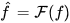is the Fourier transform of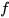and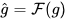is the Fourier transform of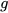. Without loss of generality, one may moreover think ofas the Fourier transform ofand ofas the Fourier transform of. We refer to the so-called "unitary, ordinary frequency" Fourier transform, i.e., its exponent includes the factor of 2 π. Choosing T = 1 and t = 0 in the first or σ = 0 in the second equation, yields the more simplified version

$$\sum_{k=-\infty}^{+\infty} f(k) = \sum_{m=-\infty}^{+\infty} \hat{f}(m)$$

which is known to be true for Schwartz functions, i.e., infinitely differentiable functions which rapidly decay to zero for large arguments.

Choosing t = 0 and T = 2 π  in the first equation, yields the formula originally found by Poisson. Choosing f = δ, the Dirac delta function, it yields the so-called distributional formulation of the Poisson Summation Formula, i.e., the equality between two Dirac combs. If the distance between two Diracs on the left is T, then the distance between two Diracs on the right becomes 1/T where T > 0 is an arbitrary real number.

The Poisson Summation Formula can be easily understood if two symbols are introduced, one for discretization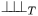and one for periodization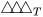. Using these symbols, the two PSF variants above become

$${\triangle \!\! \triangle \!\! \triangle}_{T} f = \frac{1}{T} \; {\cal F}^{-1}(\, {\bot \!\! \bot \!\! \bot}_{\frac{1}{T}} ({\cal F} f) \,)$$

$${\triangle \!\! \triangle \!\! \triangle}_{\frac{1}{T}} \, ({\cal F} g) = \, T \; {\cal F}({\bot \!\! \bot \!\! \bot}_{T} g).$$

They therefore represent the rules

$${\cal F}(\, {\triangle \!\! \triangle \!\! \triangle}_{T} f \,) = \frac{1}{T} \; {\bot \!\! \bot \!\! \bot}_{\frac{1}{T}} ({\cal F} f)$$

$${\cal F}({\bot \!\! \bot \!\! \bot}_{T} \, g) = \frac{1}{T} \; {\triangle \!\! \triangle \!\! \triangle}_{\frac{1}{T}} ({\cal F} g)$$

which are known as Fourier series analysis and Fourier series synthesis formula,  respectively. They moreover represent the inverse Discrete-Time Fourier Transform (IDTFT) and the Discrete-Time Fourier Transform (DTFT), respectively. For T = 1  they yield

$${\cal F}(\, {\triangle \!\! \triangle \!\! \triangle} f \,) = {\bot \!\! \bot \!\! \bot} ({\cal F} f)$$

$${\cal F}({\bot \!\! \bot \!\! \bot} \, g) = {\triangle \!\! \triangle \!\! \triangle} ({\cal F} g).$$

Hence, the Fourier transform turns periodization into discretization and discretization into periodization. In the tempered distributions sense, ifis the Dirac impulse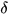then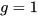is its Fourier transform and these formulas become

$${\cal F}(\, {\triangle \!\! \triangle \!\! \triangle} \, \delta) \,=\, {\bot \!\! \bot \!\! \bot} \, 1$$

$${\cal F}({\bot \!\! \bot \!\! \bot} \, 1) \,=\, {\triangle \!\! \triangle \!\! \triangle} \, \delta$$

and using the Dirac comb identity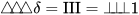it yields

$${\cal F}(\, \text{III} \,) \,=\, \text{III}$$

where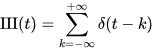is the Dirac comb. The Dirac comb identity states that it can be created either via periodizingor via discretizing the function that is constantly 1.

It can be shown that these formulas actually link four different Fourier transforms with one another, the Integral Fourier Transform, the Discrete-Time Fourier Transform (DTFT), the Discrete Fourier Transform (DFT) and the Integral Fourier Transform for periodic functions used to analyze Fourier Series. The DTFT is moreover the inverse of the Integral Fourier Transform for periodic functions. 

## References

1. C. Gasquet, P. Witomski. Fourier Analysis and Applications: Filtering, Numerical Computation, Wavelets; Marsden et.al., Eds.; Springer: New York, 1999; pp. 344.
2. Jens V. Fischer; Four Particular Cases of the Fourier Transform. Mathematics 2018, 6, 335, 10.3390/math6120335.